# Problems of the Week

Contribute a problem

# 2018-06-11 Intermediate

A plus-plus is a polygon in which all the side lengths are 1 and adjacent sides meet at right angles.

Is there a plus-plus with perimeter 2018?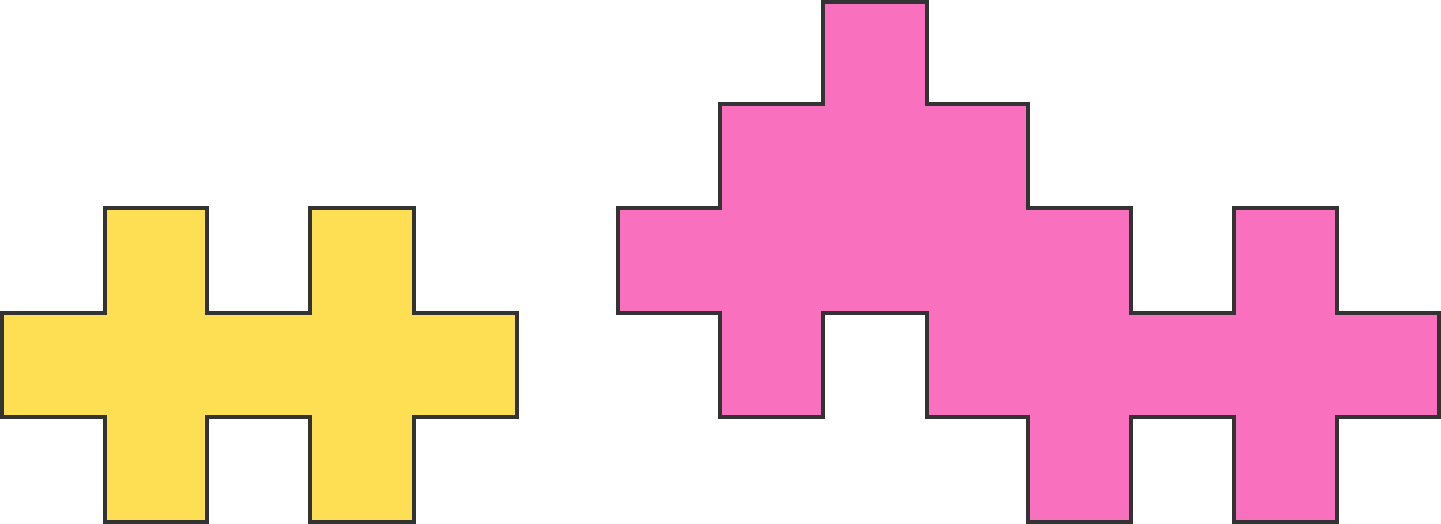Andy is unfolding a roadmap and marks each valley crease with a red dotted line and each mountain crease with a blue solid line.

Which of these patterns can he not get?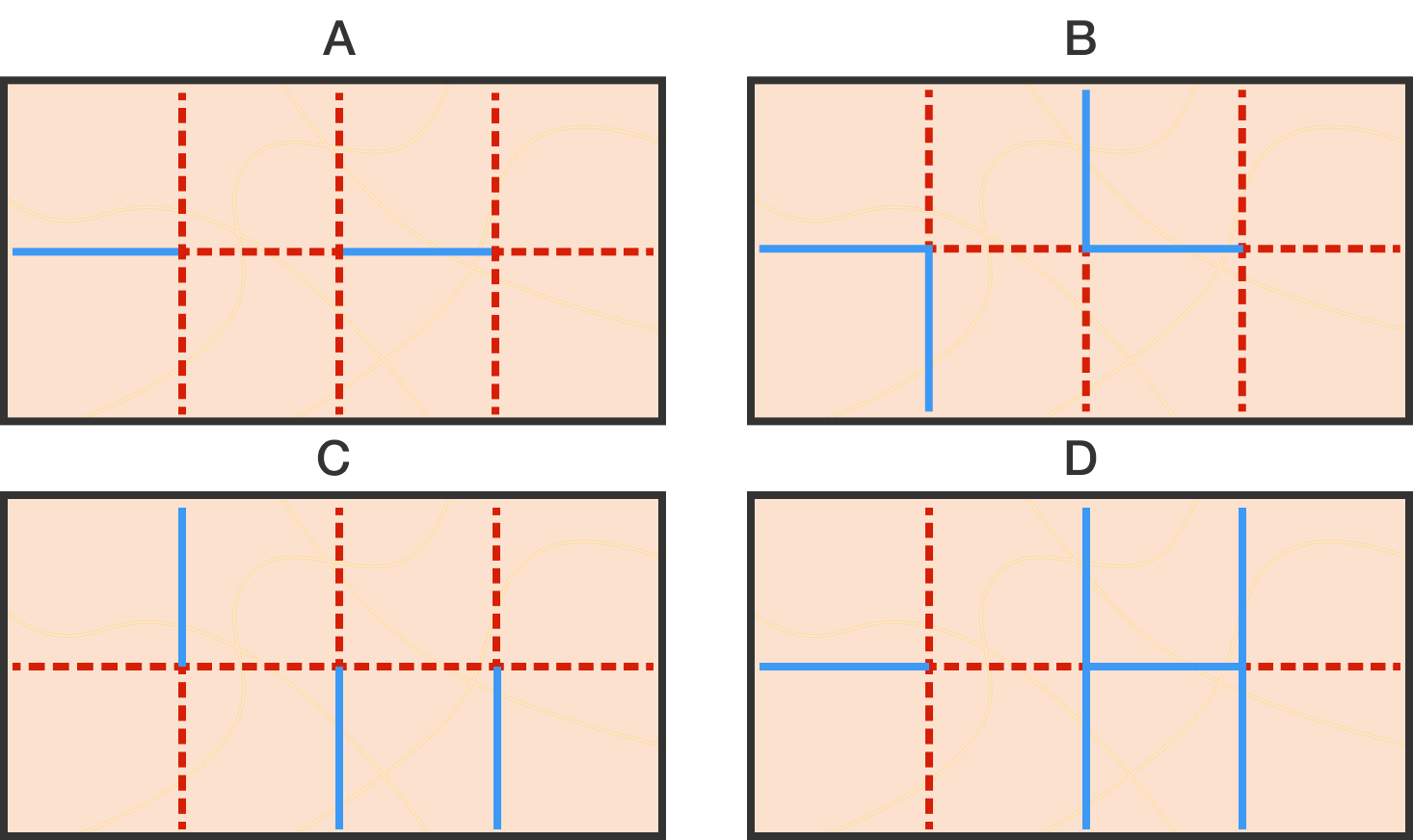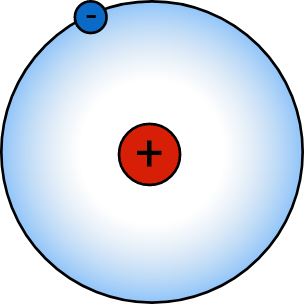Hydrogen is the simplest atom, with only one proton and one electron interacting with a Coulomb potential of $U = -\frac{e^2}{4 \pi \varepsilon_0 r}.$ If the electron were a classical charged particle, its orbit around the nucleus would decay until it came to rest at the nucleus. Since the electron is a quantum object, this doesn't happen. One way to estimate the minimum radius allowed for a quantum electron is by saturating the Heisenberg bound, which puts a lower limit on the radius of the electron's orbit $r$ and its momentum $p$: $r p \approx \Delta x \Delta p \approx \hbar.$ Treat the hydrogen atom as a two-body problem where the mass of the proton $m_p$ is much greater than that of the electron $m_e.$

Estimate the minimum radius of the electron orbit $r_\text{min}.$

Details: $e$ is the charge of an electron, $\varepsilon_0$ is the permittivity of free space, and $\hbar$ is the reduced Planck's constant.

The array 2 5 3 4 3 2 5 5 3 4 2 2 2 represents the hill below.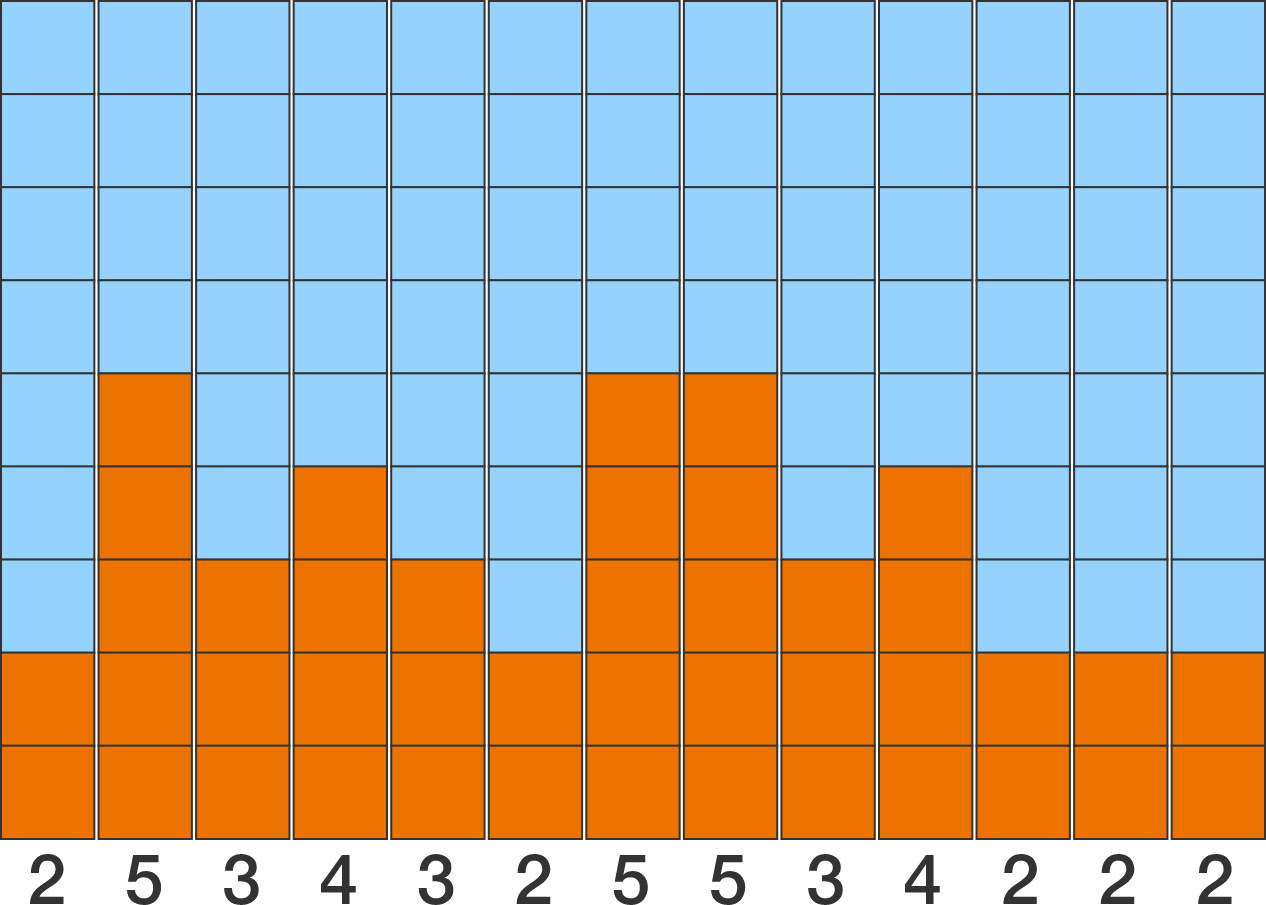If it rains in the hill, 9 units of water accumulate. (Water is assumed to run off the edges.)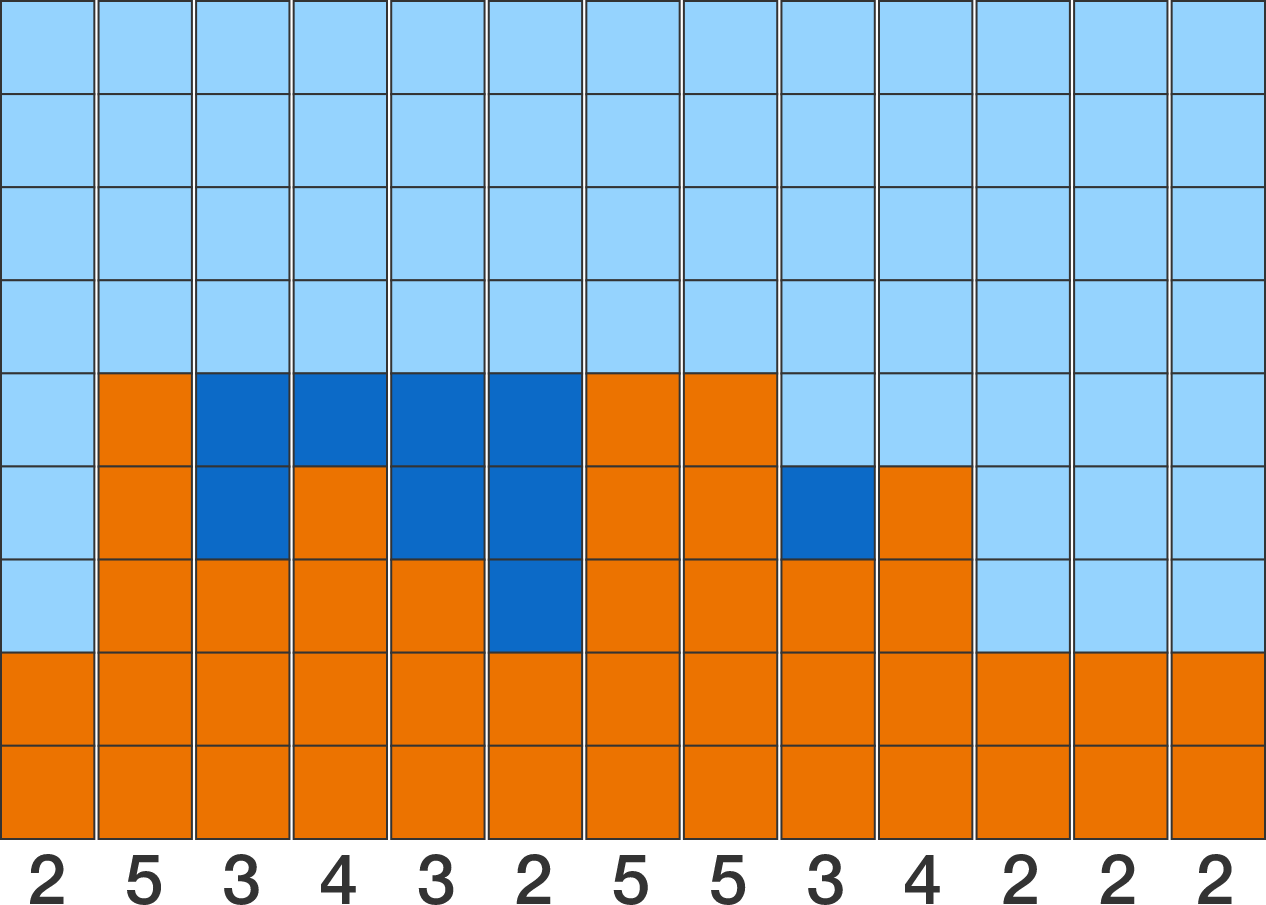Given the hill represented by the array below, how many units of water will accumulate?

hill = [4, 2, 4, 1, 5, 3, 16, 6, 17, 19, 4, 13, 5, 3, 10, 10, 13, 6, 2, 1, 5, 15,
13, 19, 16, 9, 13, 1, 7, 18, 20, 13, 9, 7, 2, 10, 8, 18, 4, 7, 5, 8, 10, 13,
7, 18, 19, 2, 19, 8, 10, 10, 17, 6, 6, 20, 20, 11, 10, 11, 13, 9, 7, 1, 10,
5, 12, 16, 10, 7, 15, 13, 12, 10, 1, 1, 4, 2, 16, 10, 20, 17, 11, 19, 19, 20,
9, 10, 17, 9, 18, 8, 10, 18, 8, 19, 16, 17, 3, 1]
You need to be connected to run code

$ABC$ is a triangle with side lengths $5,$ $4\sqrt2,$ and $7.$

Another triangle has side lengths $\sin A,$ $\sin B,$ and $\sin C.$

If the area of that triangle is $\frac{p}{q},$ where $p$ and $q$ are coprime positive integers, then what is $p+q?$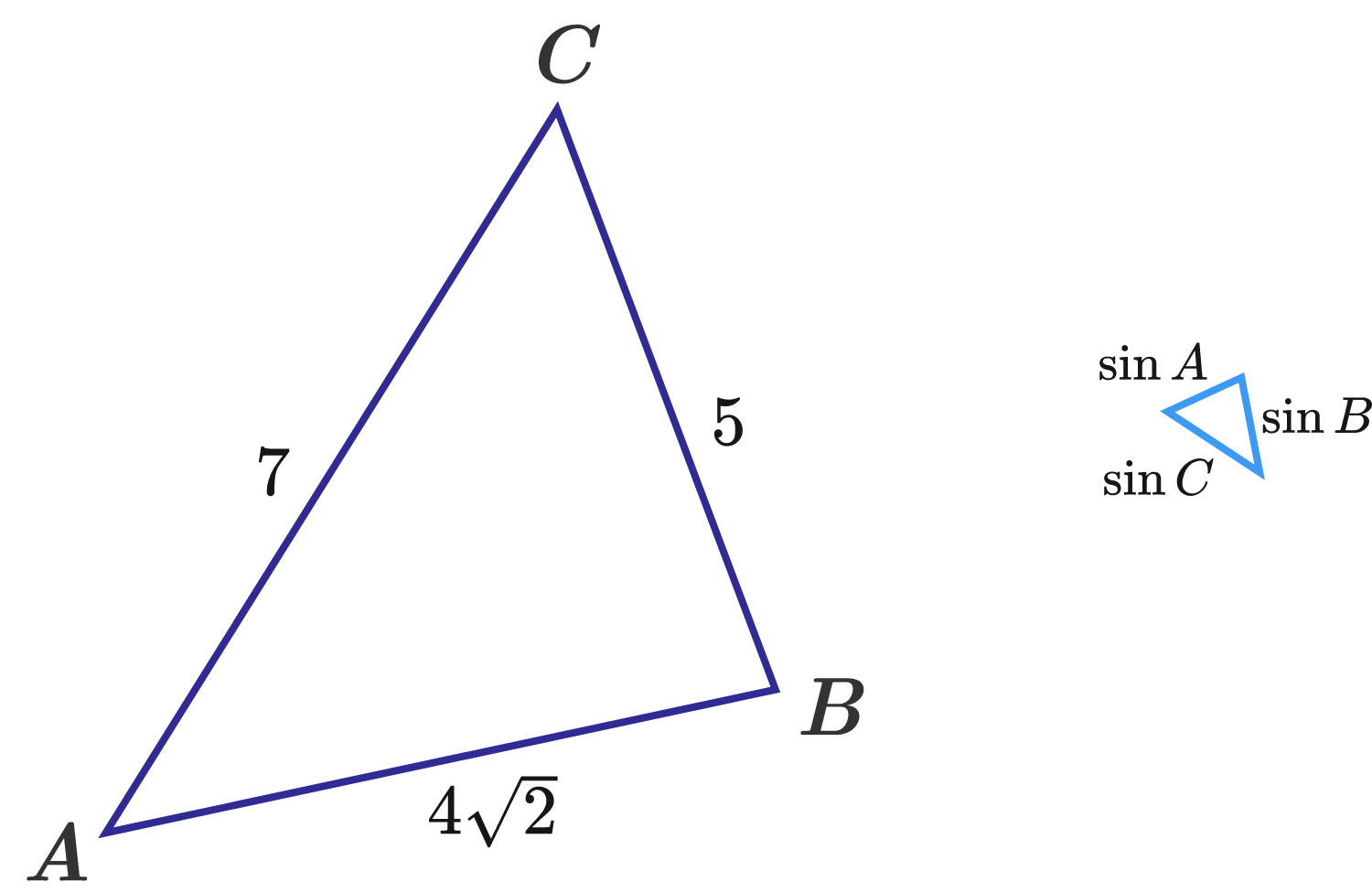×